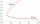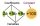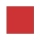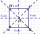# Evaluate expression

Evaluate following expression:

5 to the power of 2 - (4x4) divided by 2 =

x =  17

### Step-by-step explanation:Did you find an error or inaccuracy? Feel free to write us. Thank you!## Related math problems and questions:

• If x=4If x=4 and y=-3, what is the value of the expression x² + 2xy - 4x + 3y + y²?
• Evaluate 9Evaluate expression: 3 { 4 − 4 ( 5 − 12 ) } + 20
• Evaluate expr with 2 varsIf a=6 and x=2, find the value of (2ax + 7x- 10) /(4ax-3a-2)
• Evaluate 5Evaluate expression x2−7x+12x−4 when x=−1
• The schoolyardThe schoolyard had the shape of a square with an 11m side. The yard has been enlarged by 75 m2 and has a square shape again. How many meters was each side of the yard enlarged?
• Fractions and mixed numerals(a) Convert the following mixed numbers to improper fractions. i. 3 5/8 ii. 7 7/6 (b) Convert the following improper fraction to mixed number. i. 13/4 ii. 78/5 (c) Simplify these fractions to their lowest terms. i. 36/42 ii. 27/45 2. evaluate following ex
• The cubeThe cube has a surface area of 486 m ^ 2. Calculate its volume.
• Simplest formFind the simplest form of the following expression: 3 to the 2nd power - 1/4 to the 2nd power.
• Expression with bracketsEvaluate the expression. 15+[(9-4)×22]
• SimplifySimplify powers multiplication: (3+22)(5-42)
• Evaluate expression 2Evaluate expression with negatives: (-3)+4+(-8)+(-6)+4+(-1)
• What is 10What is the measure of the square side whose area is (4x2 + 28x+49) square units?
• Rectangular cuboidThe rectangular cuboid has a surface area 5334 cm2, and its dimensions are in the ratio 2:4:5. Find the volume of this rectangular cuboid.
• Find unknown 2Find unknown denominator: 2/3 -5/? = 1/4
• Flowerbed 2Around the square flower bed in a park is sidewalk 2 m wide. The area of ​​this sidewalk is 243 m2. What is the area of the flowerbed?
• Evaluate 12Evaluate and simplify 9 divided by 7/6.
• Fraction + eqSolve following simple equation with fractions: -5/6(8+5b) = 75 + 5/3b